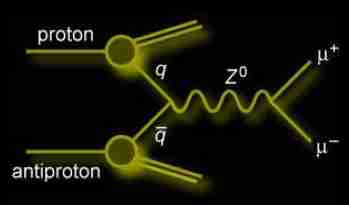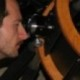## Initial and final state radiation in Z production March 14, 2007

Posted by dorigo in physics, science.

The diagrammatic way of picturing reactions between elementary particles invented by Feynman a million years ago is to theorists a powerful computational tool to derive the probability for these reactions to happen, and to experimentalists a simple, intuitive way of describing what is really happening in a particle collision, and determining what one can expect to see downstream of it.

Experimental physicists usually stop at “tree level” when drawing Feynman diagrams: they do not deal as theorists do with all the smaller and smaller corrections to the rate of a process yielded by so-called “higher order” diagrams which involve the loop of more and more virtual particles, but just want to define clearly what is the initial state of a collision (i.e., what are the projectiles flying around before a hard interaction occurs) and what is the final state (i.e., what is eventually flying out of the interaction region).

Take the following picture as an example: a simple photon-mediated interaction between two electrons (electron scattering) can be dissected by considering all diagrams involving one internal loop, as seen on the right.In these diagrams (take the bigger one as a reference) time is flowing from bottom to top (the y axis), and the two electrons labeled 1,2 move in the spatial coordinate (the x axis) coming closer until they bounce off one another a photon – described by the wiggle. [More often, as below, time and space are switched, and time goes forward in the x coordinate, space is in the y axis].

To compute the exact probability of the process, given the initial momenta and spin vectors of the colliding electrons, you do need the diagrams on the right (and if you can, even more complicated ones involving two or three loops): but to understand what is going on, the picture on the left – the “tree level” description – is perfectly adequate.

Even tree-level diagrams, however, carry their own subtleties. Let us take the “simple” process of a proton-antiproton collision at the Tevatron when two quarks annihilate creating a Z boson, which subsequently decays into a pair of opposite-charged muons. You can well picture the event with the following diagram:This is a very descriptive picture: the proton is a line moving forward in time, and so is the antiproton. But then, the two get “resolved in a blob of quarks, and two of them – a quark from the proton and an antiquark from the antiproton – interact by creating the Z, which lives a little, wiggling around until it creates the muons, which leave the interaction region by moving away from each other. In the visual simplicity of the description lies only part of the power of Feynman diagrams (their other virtue, their value as computational tools, I will not discuss here).

Now, what I really wanted to discuss in this post is how to describe a process like the one depicted above, when – together with the Z boson signal of two opposite-charged muons – you observe in your detector a high-energy photon. I discussed a process of that kind  a few days ago  in a post where I showed an event display of the D0 collaboration: in it, along with an electron-positron pair produced by the Z decay, the two additional muons were the result of the materialization of a photon: that event was indeed labeled as “associated Z-gamma production”.

So, where is this extra photon coming from ?

In a collision yielding a Z boson, the extra photon can be the result of either of two mechanisms: initial state radiation or final state radiation. They are indeed quite different processes, as I hope I can clarify with a graph:Here, the quarks on the left give rise to the Z boson, which decays to a pair of electrons on the right. The first diagram shows a photon (labeled “g”) emitted by the quark lines, while the second shows a photon emitted by the electron lines.

The description of production of a “Z-gamma” event at tree level at the Tevatron is specified by these two graphs. Experimental physicists need both of them to discuss what they expect to see in their detectors when they look for the process.

An interesting question is, what the heck is the difference between these two diagrams ? After all, from an experimental standpoint, should we not be concerned only with what was shot at what (the two interacting quarks on the left) and what came out of the collision (the three lines on the right) ?

The two diagrams are quite different in fact, and they do describe very different physical processes! The first one describes initial state radiation in Z production: the creation of a Z boson together with a radiated photon. The second one describes the creation of a Z boson and the subsequent final state radiation of a photon off the Z decay products.

So, quantum-mechanically, the two processes are distinct. But can experimentalists distinguish them ? Of course they can – and that is why each of the two diagrams above has individual value. Look at the following plot, which is real data collected by the CDF collaboration in Run II:What you see here is a “scatterplot”: data containing an electron pair and a photon is projected on two variables. The x axis is the invariant mass of the electron pair, and the y axis is the invariant mass of the whole system of three bodies.

When the Z is produced, the two electrons must have an invariant mass close to 91 GeV. That is what you see from the cluster of crosses spanning vertically the region where x (M(ee), the mass of the electron pair) is close to 91 GeV. These events, labeled “ISR” in the plot, are ones in which the photon was emitted by the quark lines, and thus the addition of its energy to the electron-positron pair makes a total invariant mass larger than 91 GeV, making a vertical spread along y.

Final state radiation events are instead labeled “FSR”: they span the region for which the total three-body mass is 91 GeV horizontally: the value of x, the dielectron mass, is smaller than 91 GeV for these events, because the two electrons we measure in the detector have lost energy to the radiated photon, and the invariant mass they make is in deficit with respect to what they had at the Z decay point, before they radiated the photon.

Together with these two processes, the plot also contains crosses spreading along a diagonal line, and labeled “DY”: these events, which we call more generically “Drell-Yan production”, are ones when the Z was not on its mass-shell: for them, one cannot distinguish whether the photon which accompanies the electron-positron pair came from the initial or the final state by energetic considerations alone. However, DY events are still completely described, at tree level, by the two diagrams shown for Z production above, provided the “Z” labeled dashed lines are replaced by “Z/gamma*”, indicating that the created particle is a superposition of these two states.1. Fred - March 15, 2007

Tommaso,
Thanks for the nice presentation. The graphics were very helpful in understanding your lesson in class today. Where can we apply to Dorigo State University? Is it an accredited institution? Do you offer scholarships for members of the golf team?
Class of ’12 ?2. dorigo - March 15, 2007

Hi Fred,

I am glad you liked the post…

The whole point of the thing was to show one scatterplot of CDF data which, in my opinion, stands out of the ordinary.

In fact, when one deals with subnuclear processes, which interfere quantum-mechanically as a natural result of their being based on complex amplitudes, one very seldom sees such a clear distinction between the contributing elements in a two-dimensional graph.

Cheers,
T.3. Alejandro Rivero - March 15, 2007

The process I like more is the impossible one: electron and antielectro, or quark and antiquark, doing the triangle into two photons.4. dorigo - March 16, 2007

Hi Alejandro,

what fascinates you of triangular ee->gg diagrams ? I never thought of those things, they of course violate a ton of rules.

T.

Sorry comments are closed for this entry